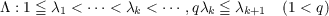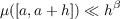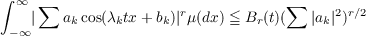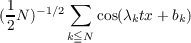#### Vol. 36, No. 1, 1971

 Download this articleFor screen For printingRecent Issues Vol. 325: 1 Vol. 324: 1  2 Vol. 323: 1  2 Vol. 322: 1  2 Vol. 321: 1  2 Vol. 320: 1  2 Vol. 319: 1  2 Vol. 318: 1  2Online Archive Volume: Issue:The Journal Subscriptions Editorial Board Officers Contacts Submission Guidelines Submission Form Policies for Authors ISSN: 1945-5844 (e-only) ISSN: 0030-8730 (print) Special Issues Author Index To Appear Other MSP Journals
Lacunary series and probability

### Robert P. Kaufman

Vol. 36 (1971), No. 1, 195–200
##### Abstract

In this note we continue some investigations connecting a lacunary series Λ of real numbersand a probability measure μ on (−∞,) satisfying(1)

for all intervals [a,a + h] of length h < 1, and a fixed exponent 0 < β < 1. (The notation X Y is a substitute for X = 0(Y ).) Measures μ occur in the theory of sets of fractional Hausdorff dimension.

In the following statements S is a subset of (−∞,) of Lebesgue measure 0, depending only on μ and Λ.

Theorem 1. For r = 2,4,6, and tS, there is a constant Br(t) so thatHere Br(t) is independent of the sequences (aj) and (bk).

Theorem 2. For tS the normalized sumstend in law (with respect to the probability μ) to the normal law. Here the convergence is uniform for all sequences (bk).

Primary: 60.30
##### Milestones